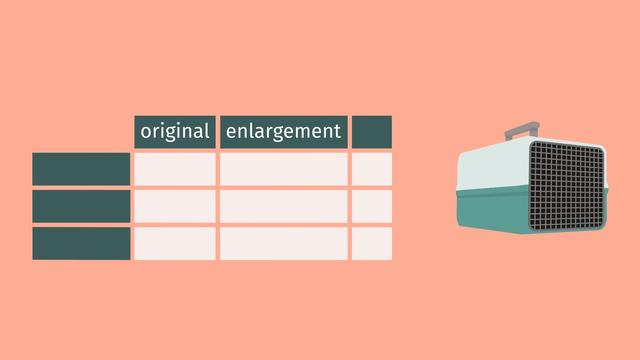# The Unit Rate as the Scale FactorRating

Ø 5.0 / 1 ratings
The authorsChris Reger

## Basics on the topicThe Unit Rate as the Scale Factor

After this lesson you will be able to calculate the scale factor in a scale drawing, and create a scale drawing with a given scale factor.

The lesson begins by teaching you that a scale factor is needed to make an enlargement. It leads to calculating unit rates and determining if the unit rates can be used as a scale factor. It concludes with using a scale factor to make a reduction.

Learn about using unit rates as scale factors by helping Junior get his giant rat into a carrying case!

This video includes key concepts, notation, and vocabulary such as: scale factor (also known as the constant of proportionality, is the ratio of any length in the scale drawing to the corresponding length in the actual picture); ratio (a comparative, proportional relationship between two amounts); reduction (a scaled-down picture of the original image); and enlargement (a scaled-up picture of the original image).

Before watching this video, you should already be familiar with equivalent ratios, recognizing if a fractional scale factor is greater than or less than 1, and multiplication and division involving fractions.

After watching this video, you will be prepared to learn how to find missing dimensions in a scale drawing, given the scale factor and dimensions of the original image.

Common Core Standard(s) in focus: 7.RP.A.2b A video intended for math students in the 7th grade